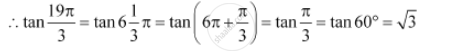# Find the Value of the Trigonometric Function Tan (19pi)/3 - Mathematics

Find the value of the trigonometric function tan  (19pi)/3

#### Solution

It is known that the values of tan repeat after an interval of π or 180°.Is there an error in this question or solution?
Chapter 3: Trigonometric Functions - Exercise 3.2 [Page 63]

#### APPEARS IN

NCERT Class 11 Mathematics
Chapter 3 Trigonometric Functions
Exercise 3.2 | Q 8 | Page 63

Share